# How to Create a Wall Street Journal Data Visualization in R

We know that the NY Times data visualizations are pretty awesome, but the Wall Street Journal’s data visualizations are nothing to laugh at. In fact, one of my favorite books on data visualization is by Dona Wong, the former graphics editor of Wall Street Journal and a student of Ed Tufte at Yale (and yes, she was at the NY Times too).

# The Wall Street Journal Data Visualization

One of my favorite data visualizations from the WSJ is the graphic on Chick-fil-A and the public opinion on same-sex marriage. The WSJ published this graphic in an article after the Chick-fil-A president commented against same-sex marriage. The author of this article suggested that Chick-fil-A could afford to express such sentiments because their stores, hence customers, were in regions with majority of the public’s opinion was against same-sex marriage.What I liked about the data visualization:

• The graphic itself was very neutral in its color palette and in its conclusions. It left it up to the readers to draw any conclusions.
• The store counts in each state were easy to see and compare. There was no cute icon for each store.
• It removed all the distractions from the data visualization. No extraneous colors, no 3-d effects, no unnecessary data.
• The colors of various regions were matched by the colors of the legends for the pie charts.
• Yes, pie charts:
• They were suitable to explain the three opinion categories.
• They all started with “Favor” at 12 o’clock.
• They were not exploding or were in 3-D, nor did they have 50 tiny slices.
• The legends were printed only once.
• A reader could quickly distinguish the public opinion slices using the simple colors.

# WSJ Data Visualization in R

Like my previous post on NYT’s data visualization, I wanted to recreate this WSJ data visualization using R. Here’s how I tried to replicate that graphic:

```library(rvest) library(stringr) library(dplyr) library(ggplot2) library(scales) library(ggmap) library(readr) library(tidyr) library(zipcode) library(maps) library(jsonlite) library(grid) library(gridExtra) data(zipcode) ## load zipcode data in your workspace```
• `rvest` to scrape data from the web
• `stringr` for string manipulation
• `dplyr` for data manipulation
• `ggplot2` for plotting, duh!
• `scales` to generate beautiful axis labels
• `ggmap` to get and plot the United States map
• `readr` to read data from CSVs
• `tidyr` to transform data from long to wide form and vice versa
• `zipcode` to clean up zipcodes and get the coordinates for each zipcode
• `jsonlite` to get data from a json object
• `grid` to line up charts
• `gridExtra` because stackoverflow said so 🙂

## Get Chick-fil-A locations

I found out that Chick-fil-A listed all its store locations on this page by each state. Every state has a separate page with store locations from that state.

So, first, I got all the states with a store in an XML document:

`states <- read_html("https://www.Chick-fil-A.com/Locations/Browse")`

Then, using the CSS selector values, I parsed the XML to extract all the hyperlink text with the URL to each state:

```locations <- states %>% html_nodes("article ul li a") %>% html_attr("href")```

You can use Selector Gadget to find CSS selectors of objects on a given web-page. Please do read rvest’s documentation for more information on using `rvest` for web-scraping.

You will get a character vector of all the relative urls of each state. Like this:

``##  "/Locations/Browse/AL" "/Locations/Browse/AZ" "/Locations/Browse/AR"``

So, we need to join this relative URL to the top domain:

`locations <- paste0("https://www.Chick-fil-A.com", locations)`

Now, we need to scrape every state page and extract the zipcode of all the stores on that page. I wrote a function to help us with that:

```extract_location <- function(url){ read_html(url) %>% html_nodes(".location p") %>% html_text() %>% str_extract("\\d{5}(?=\n)") }```

The function will download each URL, find the `div` with the `location` class, convert the match to text, and lastly extract the five digit number (zipcode) using regular expression.

We need to pass the URL of each state to this function. We can achieve that by using `sapply` function, but be patient as this will take a minute or two:

`locationzips <- sapply(locations, extract_location, USE.NAMES = FALSE)`

## Clean up the store zip

To make the above data usable for this data visualization, we need to put the zipcode list in a data frame.

`locationzips_df <- data.frame(zips = unlist(locationzips), stringsAsFactors = FALSE)`

If this post goes viral, I wouldn’t want millions of people trying to download this data from Chick-fil-A’s site. I saved you these steps and stored the zips in a csv on my dropbox (notice the `raw=1` parameter at the end to get the file directly and not the preview from dropbox):

`locationzips_df <- read_csv("https://www.dropbox.com/s/x8xwdx61go07e4e/chickfilalocationzips.csv?raw=1", col_types = "c")`

In case we got some bad data, clean up the zipcodes using `clean.zipcodes` function from the `zipcode` package.

`locationzips_df\$zips <- clean.zipcodes(locationzips_df\$zips)`

## Merge coordinate data

Next, we need to get the coordinate data on each zipcode. Again, the dataset from the `zipcode` package provides us with that data.

`locationzips_df <- merge(locationzips_df, select(zipcode, zip, latitude, longitude, state), by.x = 'zips', by.y = 'zip', all.x = TRUE)`

## Calculate the total number of stores by state

This is really easy with some `dplyr` magic:

`rest_cnt_by_state <- count(locationzips_df, state)`

This data frame will look something like this:

``````## # A tibble: 6 × 2
##   state     n
##
## 1    AL    77
## 2    AR    32
## 3    AZ    35
## 4    CA    90
## 5    CO    47
## 6    CT     7``````

## Gather the public opinion data

The PRRI portal shows various survey data on American values. The latest year with data is 2015. I dug into the HTML to find the path to save the JSON data:

```region_opinion_favor <- fromJSON("http://ava.prri.org/ajx_map.regionsdata?category=lgbt_ssm&sc=2&year=2015&topic=lgbt")\$regions region_opinion_oppose <- fromJSON("http://ava.prri.org/ajx_map.regionsdata?category=lgbt_ssm&sc=3&year=2015&topic=lgbt")\$regions```

Next, I added a field to note the opinion:

```region_opinion_favor\$opi <- 'favor' region_opinion_oppose\$opi <- 'oppose'```

Then, I manipulated this data to make it usable for the pie charts:

```region_opinion <- bind_rows(region_opinion_favor, region_opinion_oppose) %>% filter(region != 'national') %>% mutate(region = recode(region, "1" = "Northeast", "2" = "Midwest", "3" = "South", "4" = "West")) %>% spread(key = opi, value = percent) %>% mutate(other = 100 - favor - oppose) %>% gather(key = opi, value = percent, -region, -sort) %>% select(-sort) %>% mutate(opi = factor(opi, levels = c('oppose', 'other', 'favor'), ordered = TRUE))```

There’s a lot of stuff going on in this code. Let me explain:

1. We bind the two data frames we created
2. We remove the data row for the national average
3. We recode the numerical regions to text regions
4. We spread the data from long format to wide format. Read tidyr documentation for further explanation.
5. Now that the opinions are two columns, we create another column for the remaining/unknown opinions.
6. We bring everything back to long form using `gather`.
7. We remove the `sort` column.
8. We create an `ordered factor` for the opinion. This is so that the oppose opinion shows up at the top on the charts.

After all that, we get a data frame that looks like this:

``````##      region    opi percent
## 1 Northeast  favor      63
## 2   Midwest  favor      54
## 3     South  favor      46
## 4      West  favor      59
## 5 Northeast oppose      29
## 6   Midwest oppose      38``````

The WSJ data visualization did one more useful thing: it ordered the pie charts with the regions with most opposition to same-sex marriage at the top. The way to handle this kind of stuff in `ggplot` is to order the underlying factors.

```regions_oppose_sorted <- arrange(filter(region_opinion, opi == 'oppose'), desc(percent))\$region region_opinion <- mutate(region_opinion, region = factor(region, levels = regions_oppose_sorted, ordered = TRUE))```

Now that our dataframe is ready, we can create the pie charts using `ggplot`.

## Create the pie charts

To create the pie charts in `ggplot`, we actually create stacked bar graphs first and then change the polar coordinates. We could also use the `base R` plotting functions, but I wanted to test the limits of `ggplot`.

`opin_pie_charts <- ggplot(region_opinion, aes(x = 1, y = percent, fill = opi)) + geom_bar(width = 1,stat = "identity", size = 0.3, color = "white", alpha = 0.85, show.legend = FALSE)`

There are few things worth explaining here:

• We set the `aes x` value to 1 as we really don’t have an x-axis.
• We set the `aes fill` value to the variable `opi` that has values of oppose, favor and other.
• We set the bar `width` to 1. I chose this value after some iterations.
• We set the `stat` to `identity` because we don’t `ggplot` to calculate the bar proportions.
• We set the `color` to white – this is the color of the separator between each stack of the bars.
• We set the `size` to 0.3 that gives us reasonable spacing between the stacks. I did so to match the WSJ visualization.

This is the chart we get: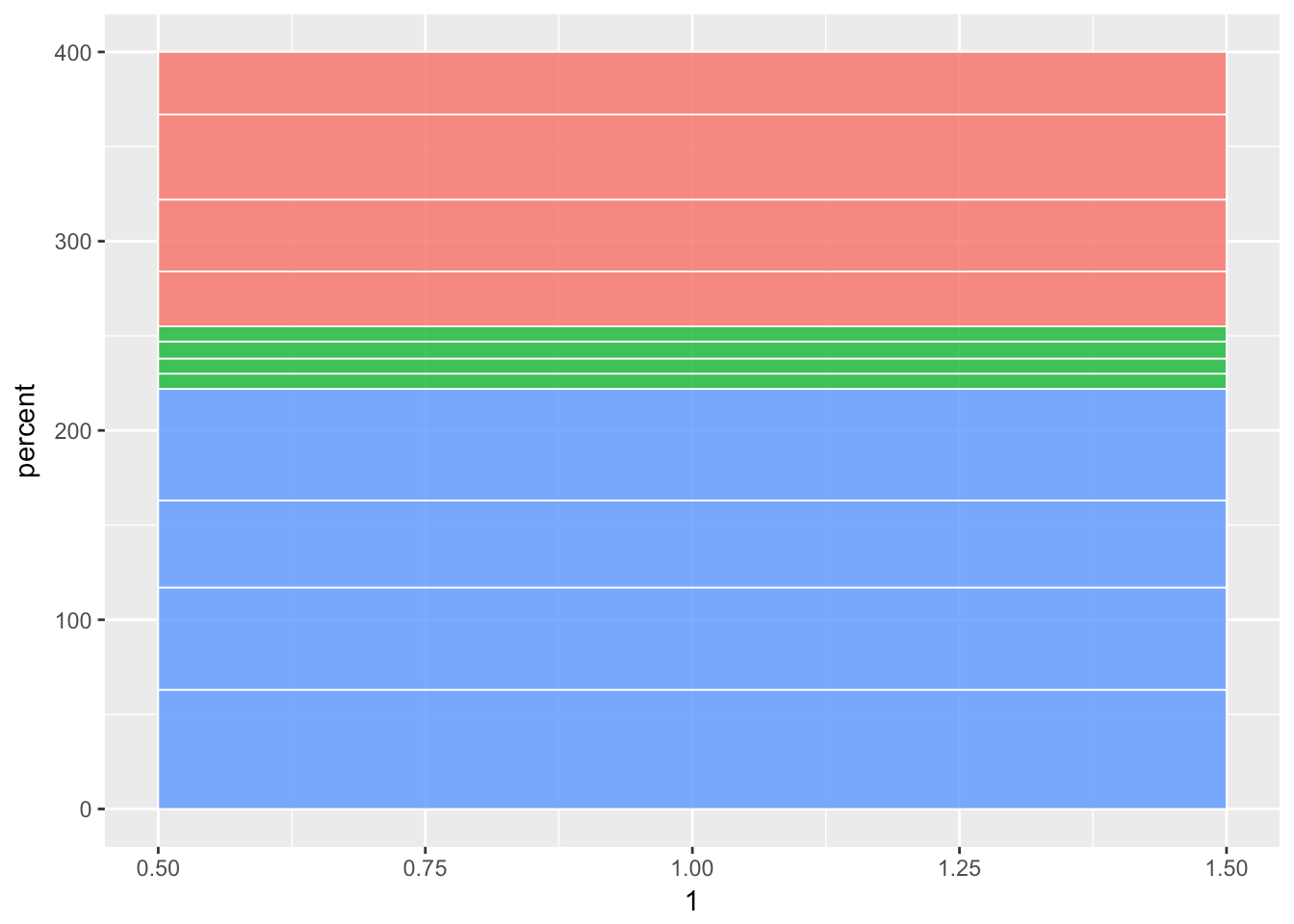And, your reaction totally may be like this:first time you see the default ggplot stacked bar colors

Well, relax, Kevin. This will get better. I promise.

Next, we add `facets` to create a separate chart for each region as well as adding the polar coordinates to create the pie chart. I also added `theme_void` to remove all the gridlines, axis lines, and labels.

`opin_pie_charts <- opin_pie_charts + facet_grid(region ~ ., switch = "y") + coord_polar("y") + theme_void()`

Resulting in this: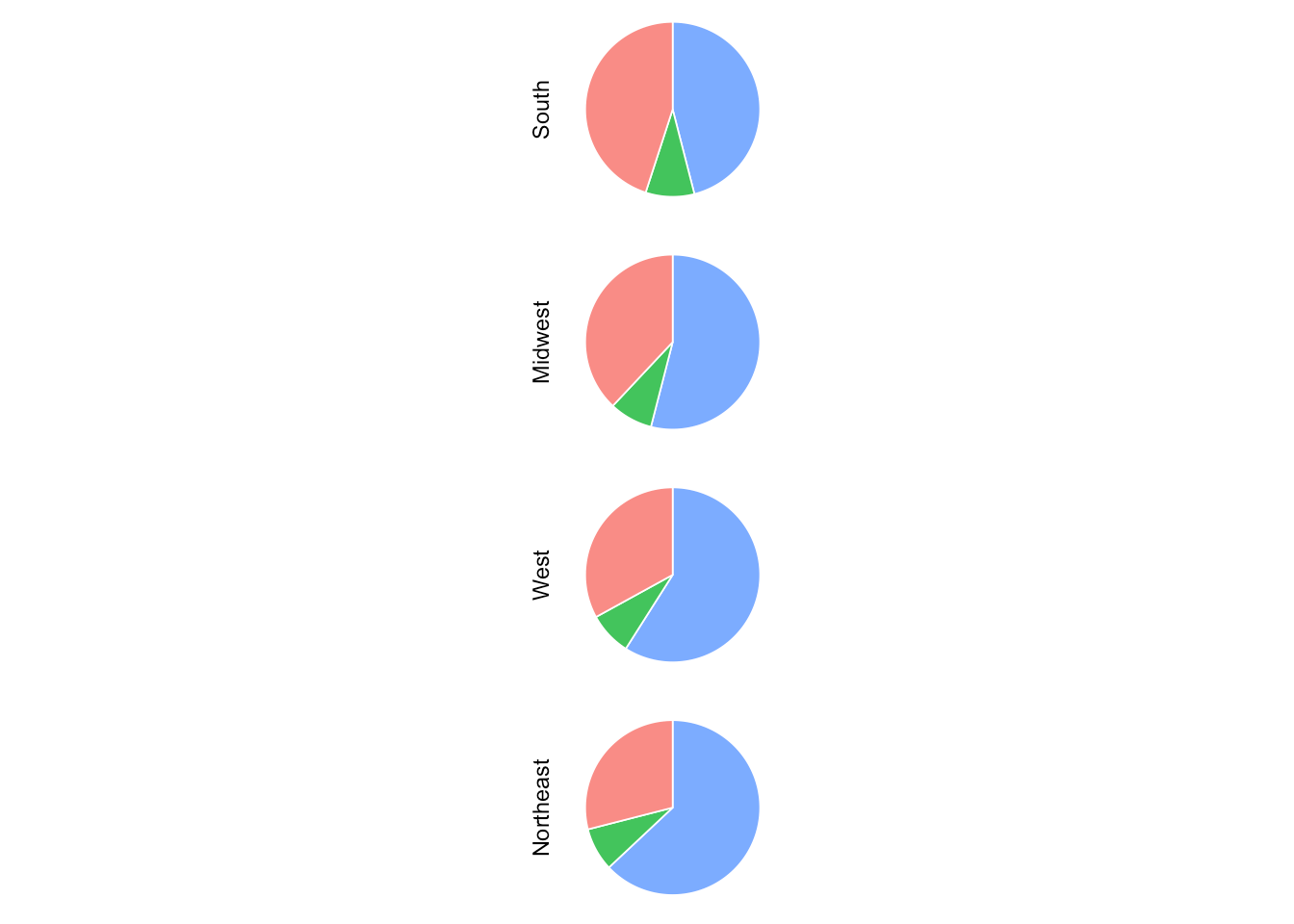Feeling better, Kevin?Let’s change the colors, which I found using image color picker, of the slices to match with the WSJ’s data visualization:

`opin_pie_charts <- opin_pie_charts + scale_fill_manual(values = c("favor" = "black", "oppose" = "#908E89", "other" = "#F7F3E8"))`

Giving us this: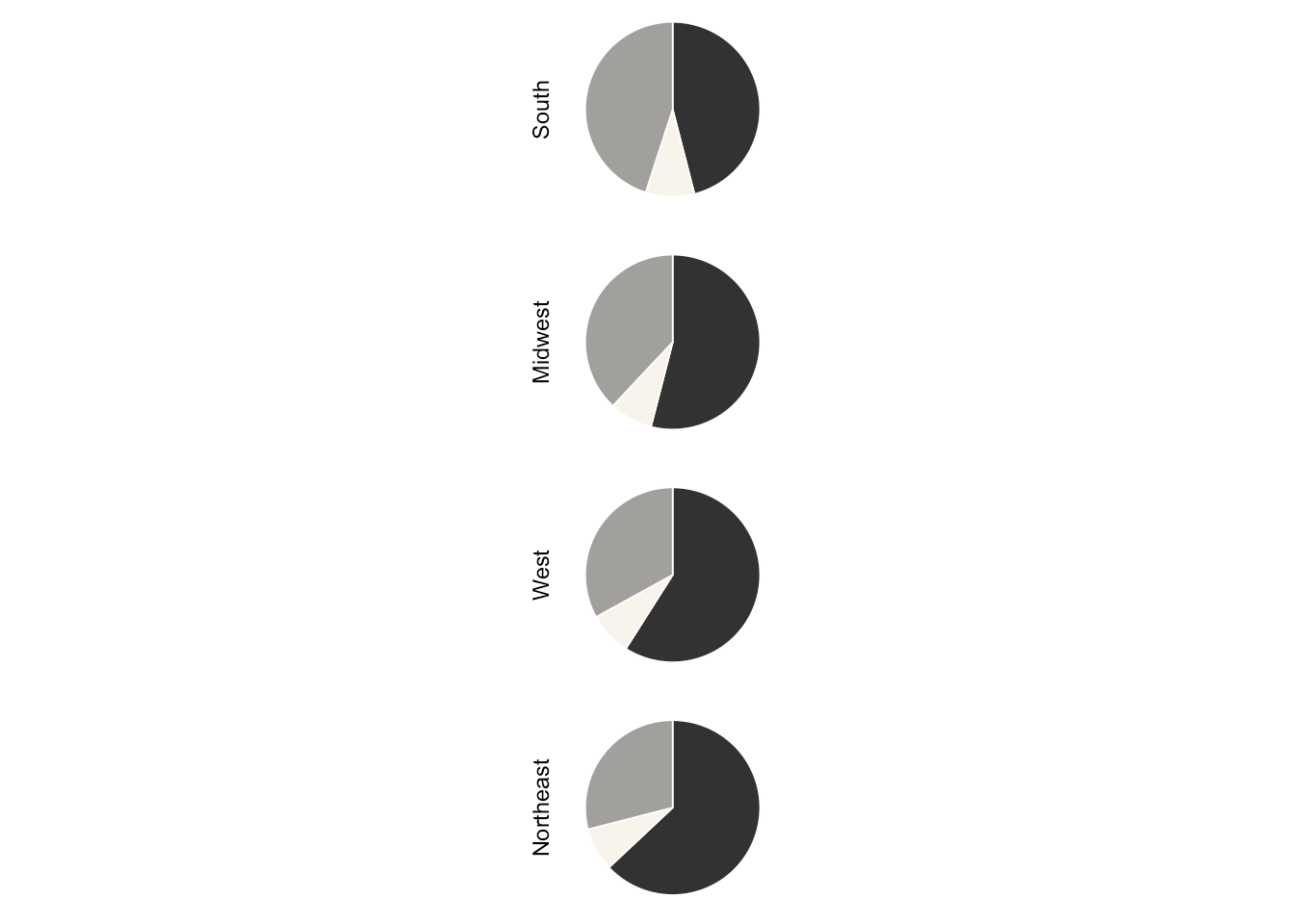Next, add data tables. I just picked some good values where it would make sense to show the labels:

```#add labels opin_pie_charts <- opin_pie_charts + geom_text(color = "white", size = rel(3.5), aes(x = 1, y = ifelse(opi == 'favor', 15, 85), label = ifelse(opi == 'other', NA, paste0(str_to_title(opi), "\n", percent(percent/100)))))```

Resulting in this: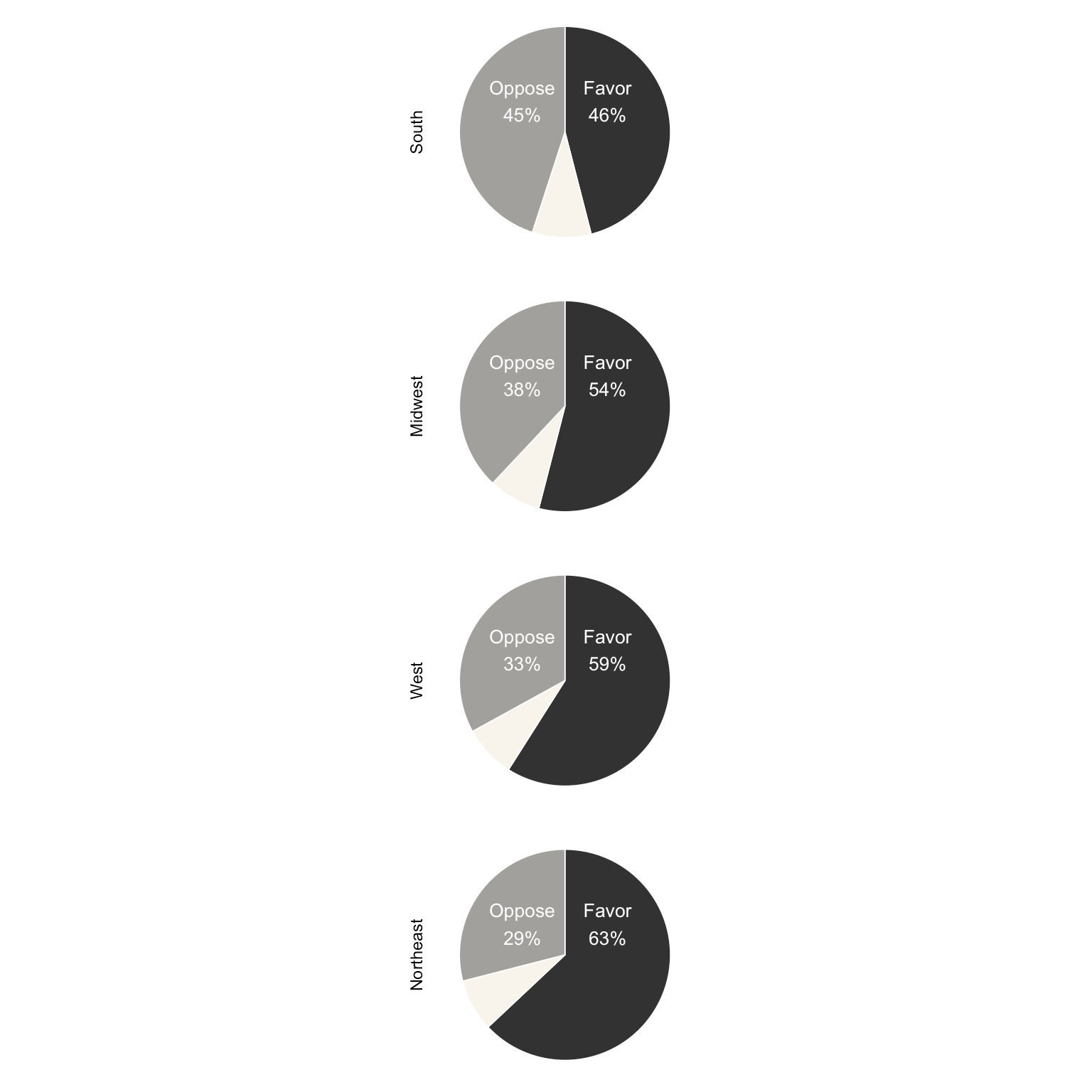Next, adding the plot title as given in the WSJ data visualization:

`opin_pie_charts <- opin_pie_charts + ggtitle(label = "Survey of views of gay\nmarriage, by region") + theme(plot.title = element_text(size = 10, face = "bold", hjust = 1))`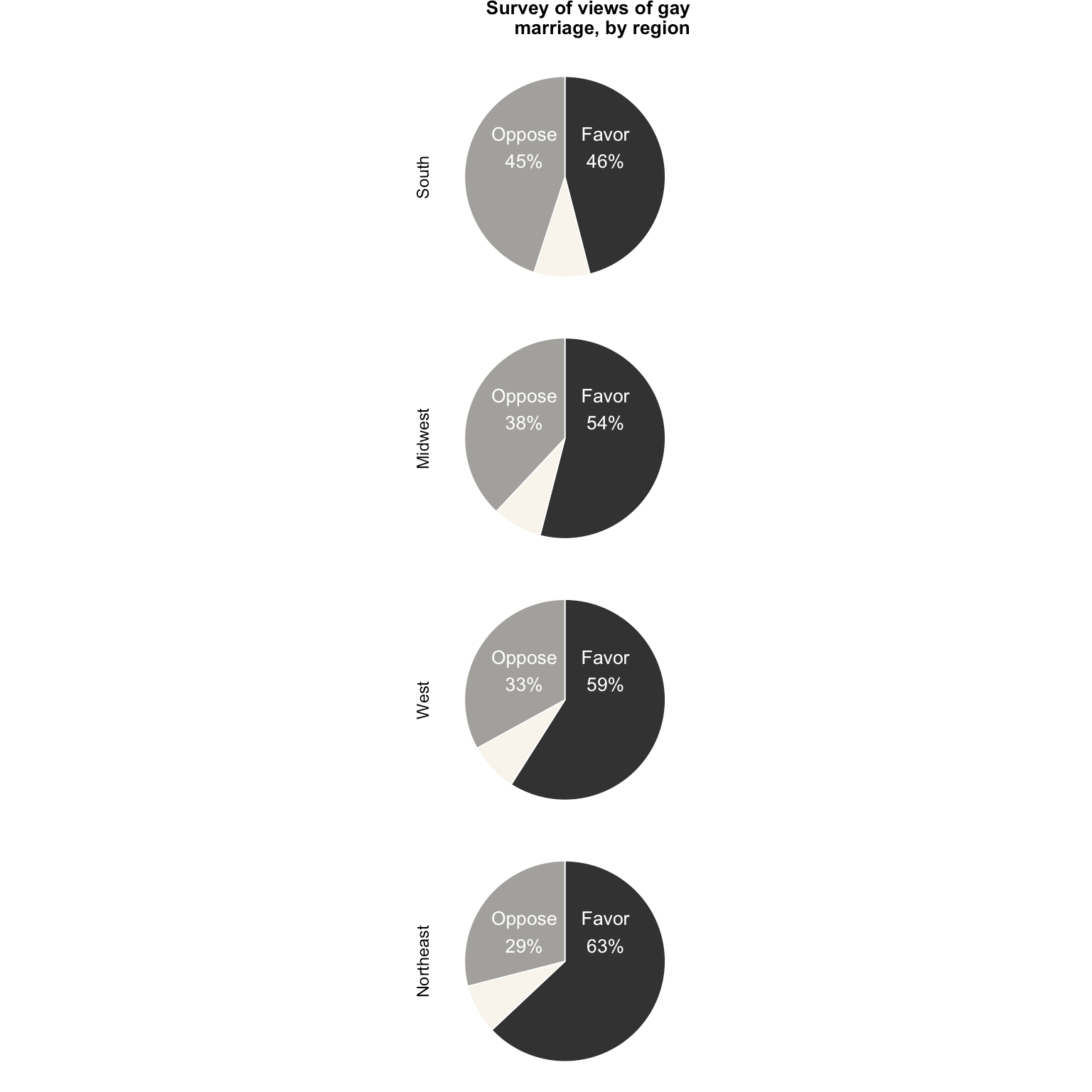And, the last step:

• change the background color of the region labels,
• emphasize the region labels
• change the panel color as well as the plot background color
`opin_pie_charts <- opin_pie_charts + theme(strip.background = element_rect(fill = "#E6D9B7"), strip.text = element_text(face = "bold"), plot.background = element_rect(fill = "#F6F4E8", color = NA), panel.background = element_rect(fill = "#F6F4E8", color = NA))`

Getting us really close to the WSJ pie charts: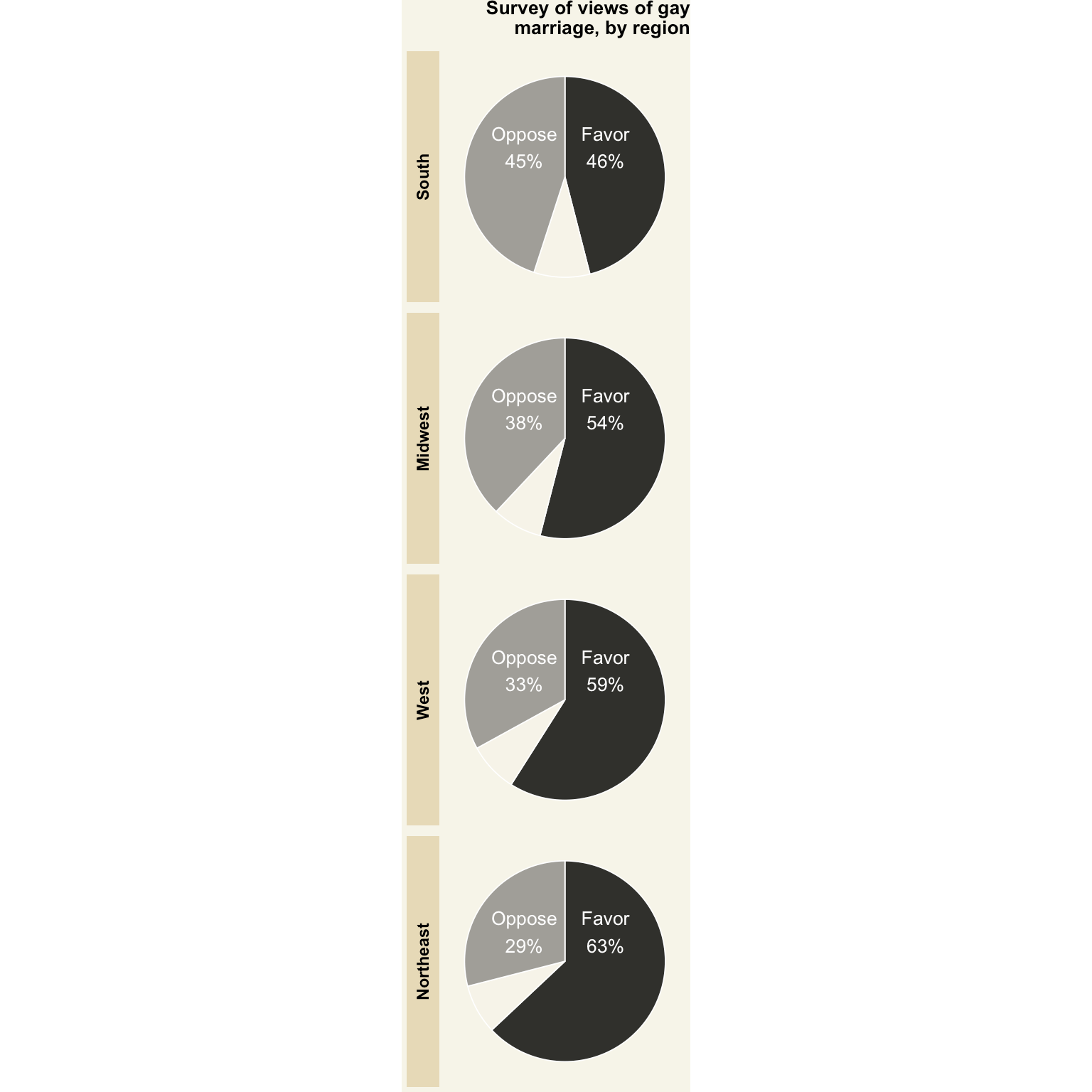I should say that a pie chart in `ggplot` is difficult to customize because you lose one axis. Next time, I would try the `base R` pie chart.

## Creating the map

Phew! That was some work to make the pie charts look similar to the WSJ data visualization. Now, more fun: the maps.

First, let’s get some data ready.

`R` comes with various data points on each of the states. So, we will get the centers of the states, abbreviations, regions, and state names.

```state_centers_abb <- data.frame(state = tolower(state.name), stateabr = state.abb, center_long = state.center\$x, center_lat = state.center\$y, region = state.region)```

Which gives us this:

``````##        state stateabr center_long center_lat region
## 1    alabama       AL    -86.7509    32.5901  South
## 2     alaska       AK   -127.2500    49.2500   West
## 3    arizona       AZ   -111.6250    34.2192   West
## 4   arkansas       AR    -92.2992    34.7336  South
## 5 california       CA   -119.7730    36.5341   West
## 6   colorado       CO   -105.5130    38.6777   West``````

The regions in this data set have a value of `North Central`; let’s change that to `Midwest`.

`state_centers_abb <- mutate(state_centers_abb, region = recode(region, "North Central" = "Midwest"))`

Next, let’s get the polygon data on each state and merge it with the state centers data frame:

```us_map <- map_data("state") us_map <- rename(us_map, state = region) us_map <- inner_join(us_map, state_centers_abb, by = c("state" = "state")) us_map <- us_map[order(us_map\$order), ]```

While plotting the polygon data using `ggplot`, you have to make sure that the order column of the polygon data frame is ordered, otherwise, you will get some wacky looking shapes.

With some prep work done, let the fun begin. Let’s create the base map:

`base_plot <- ggplot(data = left_join(us_map, rest_cnt_by_state, by = c("stateabr" = "state")), aes(x = long, y = lat, group = group, order = order, fill = region)) + geom_polygon(color = "white")`

You will note that I’ve joined the polygon data frame with the counts of restaurants in each state. Also, similar to the stacked bar graphs, I’ve separated each state with the white color, giving us this: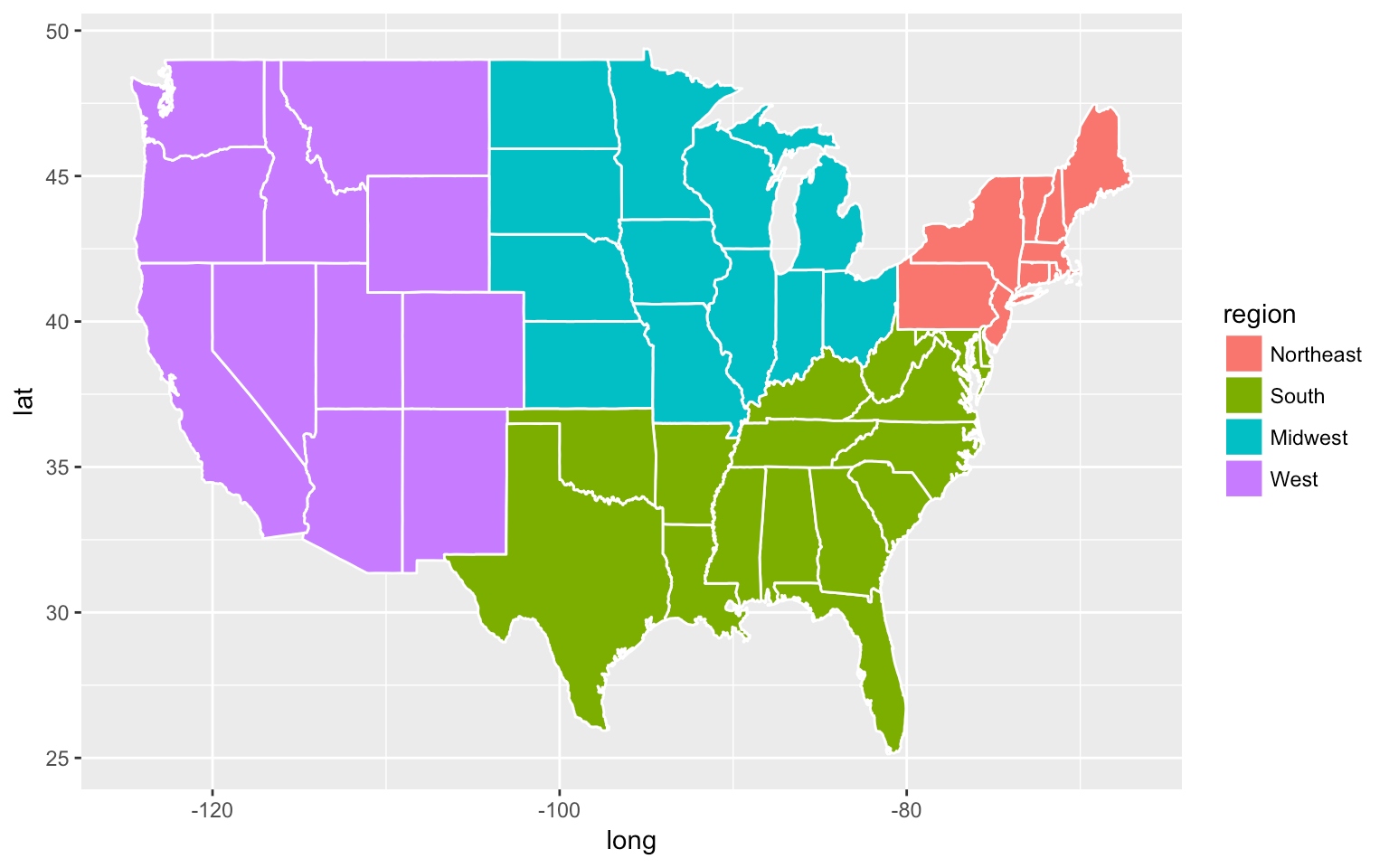I already see Kevin going crazy!

I again used the image color picker to select the colors from the WSJ data visualization and assigned them manually to each region. I also removed the legend:

`base_plot <- base_plot + scale_fill_manual(values = c("West" = "#E6D9B7", "South" = "#F2DBD5", "Northeast" = "#D8E7D7", "Midwest" = "#D6DBE3"), guide = FALSE)`

Generating this: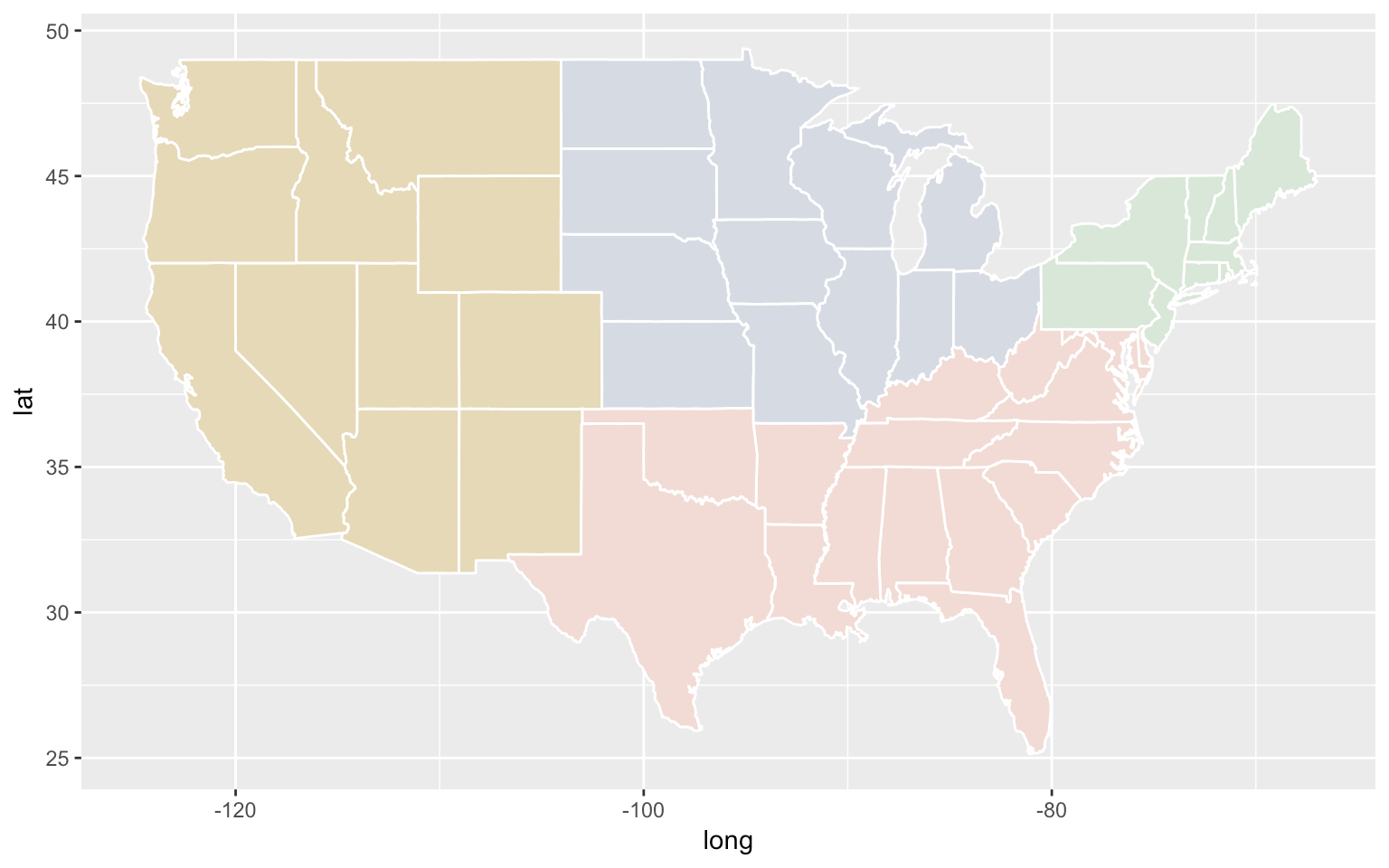Next, remove all the distractions and change the background color:

```base_plot <- base_plot + theme(axis.title = element_blank(), axis.ticks = element_blank(), axis.line = element_blank(), axis.text = element_blank(), panel.grid = element_blank(), panel.border = element_blank(), plot.background = element_rect(fill = "#F6F4E8"), panel.background = element_rect(fill = "#F6F4E8"))```

Giving us this: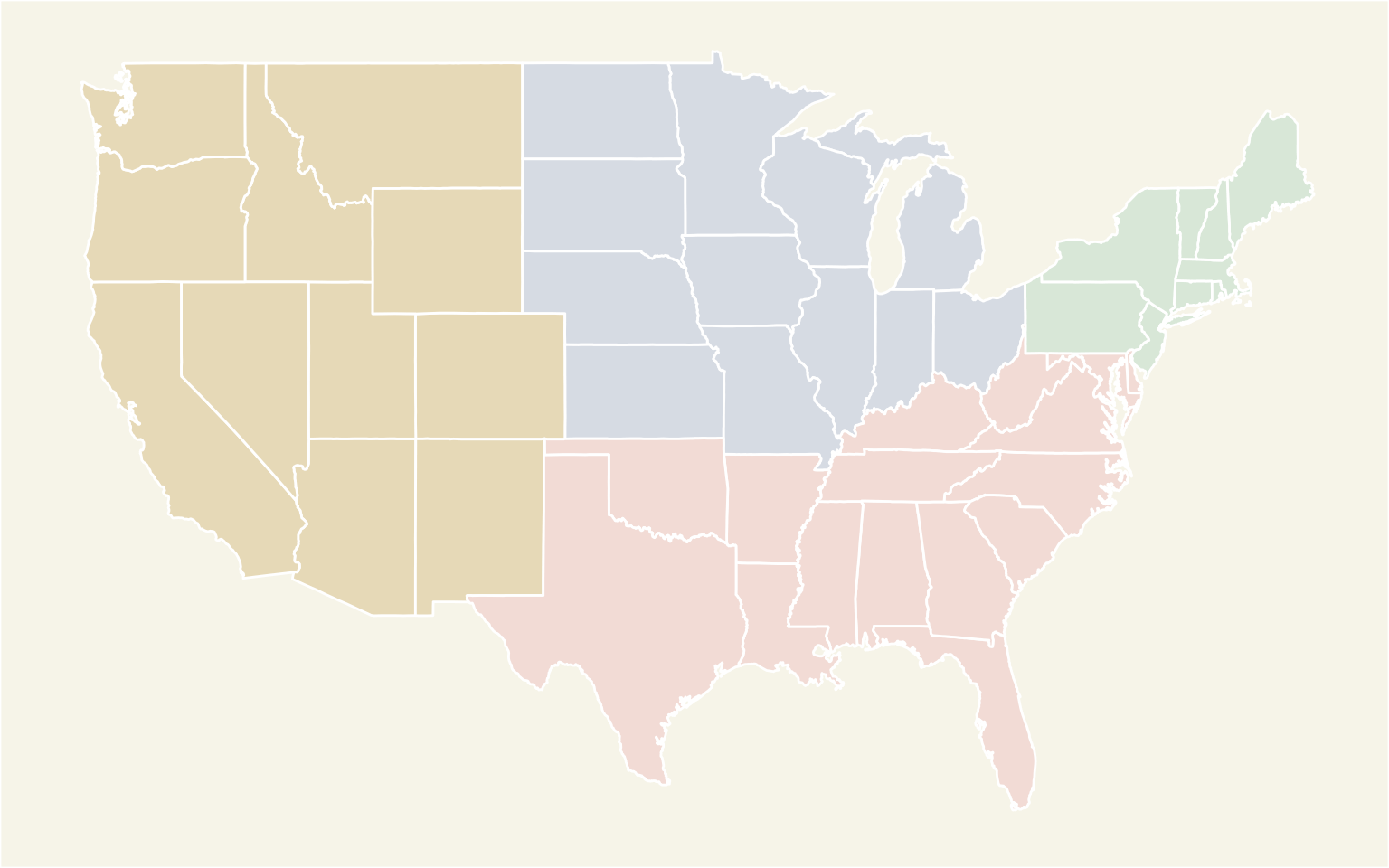Next up is adding the circles for restaurant counts.

`base_plot <- base_plot + geom_point(aes(size = n, x = center_long, y = center_lat), shape = 1, stroke = 0.5, color = "grey60", inherit.aes = FALSE) + scale_size_area(max_size = 18, breaks = c(0, 10, 100, 300), guide = FALSE) + scale_shape(solid = FALSE)`

OK. There is a lot of stuff going on here. First, we use `geom_point` to create the circles at the center of each state. The size of the circle is dictated by the number of restaurants in each state. The `stroke` parameters controls the thickness of the circle. We also are using `inherit.aes = FALSE` to create new aesthetics for this geom.

The `scale_size_area` is very important because as the documentation says:

scale_size scales area, scale_radius scales radius. The size aesthetic is most commonly used for points and text, and humans perceive the area of points (not their radius), so this provides for optimal perception. scale_size_area ensures that a value of 0 is mapped to a size of 0.

Don’t let this happen to you! Use the area and not the radius.

I also increased the size of the circles and gave breaks manually to the circle sizes.

Generating this: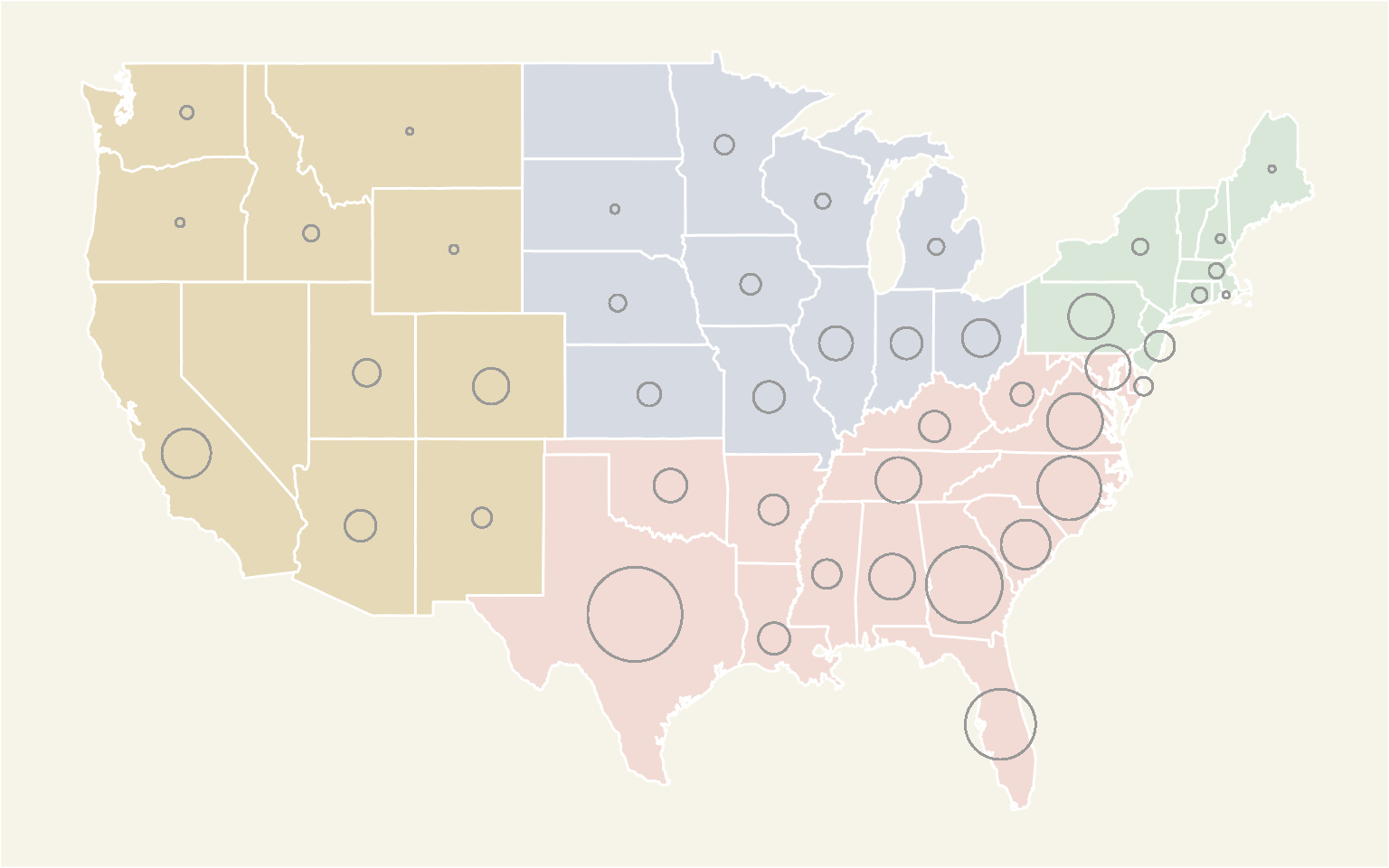Since we removed the legend for the circle size and the WSJ graphic had one, let’s try to add that back in. This was challenging and hardly accurate. I played with some sizes and eyeballed to match the radii of different circles. I don’t recommend this at all. This is better handled in post-processing using Inkscape or Illustrator.

Please add the circles as a legend in post-processing. Adding circle grobs is not accurate and doesn’t produce the desired results.
`base_plot <- base_plot + annotation_custom(grob = circleGrob(r = unit(1.4, "lines"), gp = gpar(fill = "gray87", alpha = 0.5)), xmin = -79, xmax = -77, ymin = 48 , ymax = 49) + annotation_custom(grob = circleGrob(r = unit(0.7, "lines"), gp = gpar(fill = "gray85", alpha = 0.87)), xmin = -79, xmax = -77, ymin = 47.4, ymax = 48) + annotation_custom(grob = circleGrob(r = unit(0.3, "lines"), gp = gpar(fill = "gray80", alpha = 0.95)), xmin = -79, xmax = -77, ymin = 46.5, ymax = 48)`

Giving us: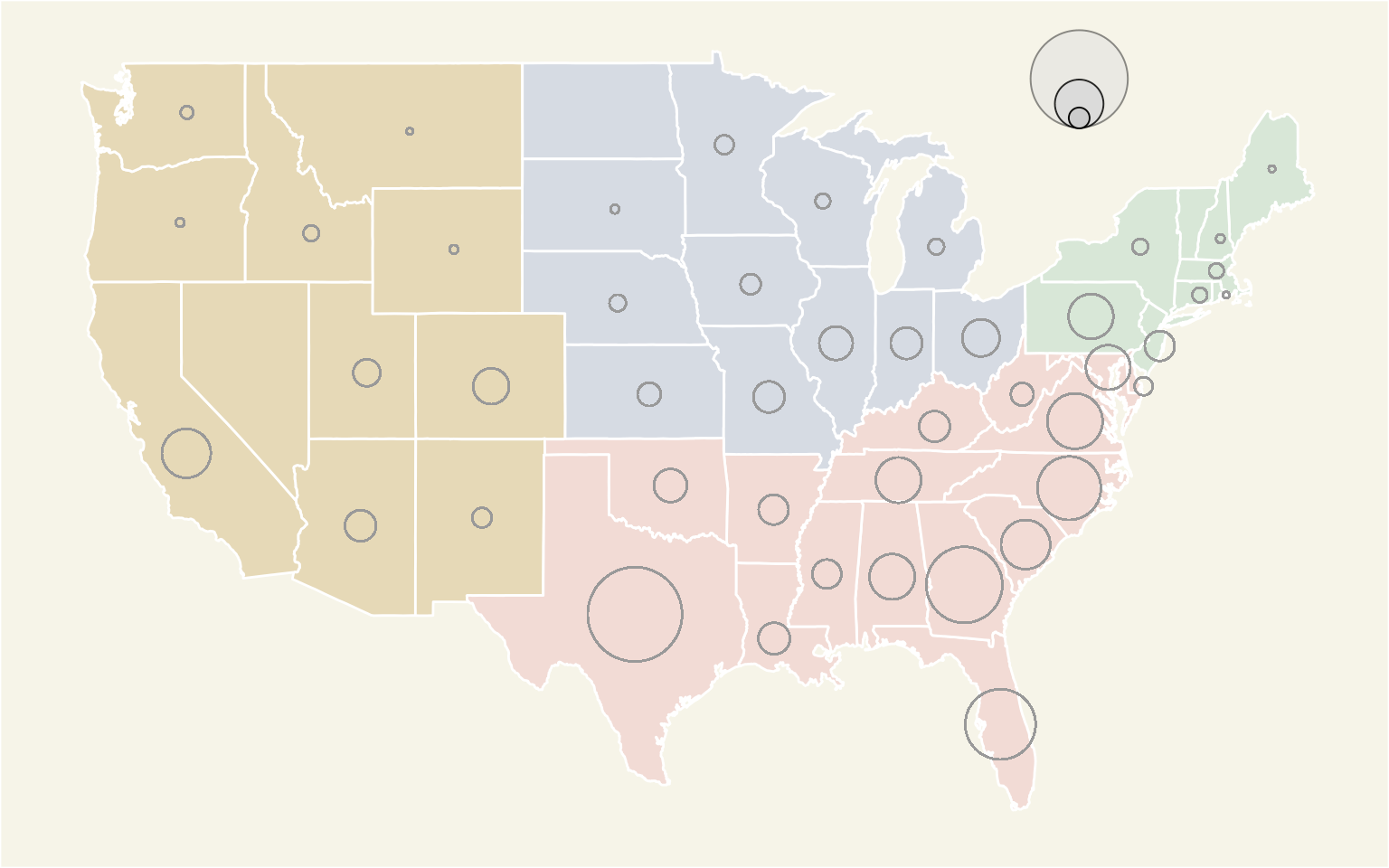`base_plot <- base_plot + ggtitle(label = "Number of Chick-fil-A resturants by state and region") + theme(plot.title = element_text(size = 10, face = "bold", hjust = 0.14, vjust = -5))`

## Merging the states and pie charts

This is the easiest step of all. We use the `grid.arrange` function to merge the two plots to create our own WSJ data visualization in R.

```png("wsj-Chick-fil-A-data-visualization-r-plot.png", width = 800, height = 500, units = "px") grid.arrange(base_plot, opin_pie_charts, widths= c(4, 1), nrow = 1) dev.off()```

Here it is: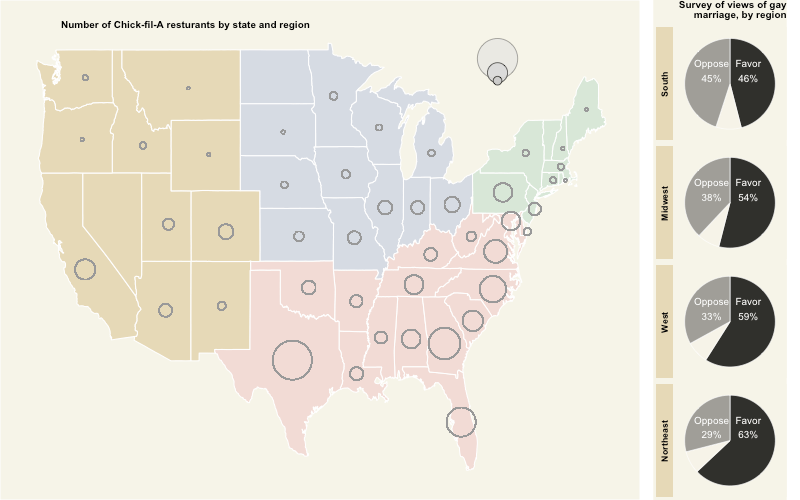What do you think?

Some things I couldn’t make work:

• Change the color of the facet of the pie charts. I toyed with `strip.text` settings, but I couldn’t change all the colors. Perhaps, it is easy to do so in the `base R` pie charts.
• The circle-in-circle legend. I got the circles, but not the numbers.
• The ‘other’ category label in the pie chart.
• Make the circles on the maps look less jagged-y.

# Does the data visualization still work?

Of course, the public opinion over same-sex marriage has changed across the states and Chick-fil-A has opened up more stores across the country.

It still does look like that bigger circles are in the regions where people oppose the same-sex marriage.

I wanted to question that. According to the Pew research center data, only 33% of Republicans favor same-sex marriage. So, if we were to plot the 2016 U.S. Presidential elections by county and plot each zipcode of Chick-fil-A stores, we can see whether there are more stores in counties voting for Donald Trump.

Let’s get started then.

Get the county level data. Tony McGovern already did all the hard work:

```cnty_results <- read_csv("https://raw.githubusercontent.com/tonmcg/County_Level_Election_Results_12-16/master/2016_US_County_Level_Presidential_Results.csv") cnty_results <- mutate(cnty_results, per_point_diff = per_gop - per_dem, county_name = tolower(str_replace(county_name, " County", "")), county_name = tolower(str_replace(county_name, " parish", "")))```

Get the county map polygon data:

```county_map <- map_data("county") county_map <- rename(county_map, state = region, county = subregion) county_map\$stateabr <- state.abb[match(county_map\$state, tolower(state.name))]```

Join the polygon with county results:

`cnty_map_results <- left_join(county_map, cnty_results, by = c("stateabr" = "state_abbr", "county" = "county_name")) %>% arrange(order, group)`

Plot the results and counties:

`cnty_plot <- ggplot(data = cnty_map_results, aes(x = long, y = lat, group = group, order = order)) + geom_polygon(aes(fill = per_point_diff), color = "gray90", size = 0.1)`

This what we get: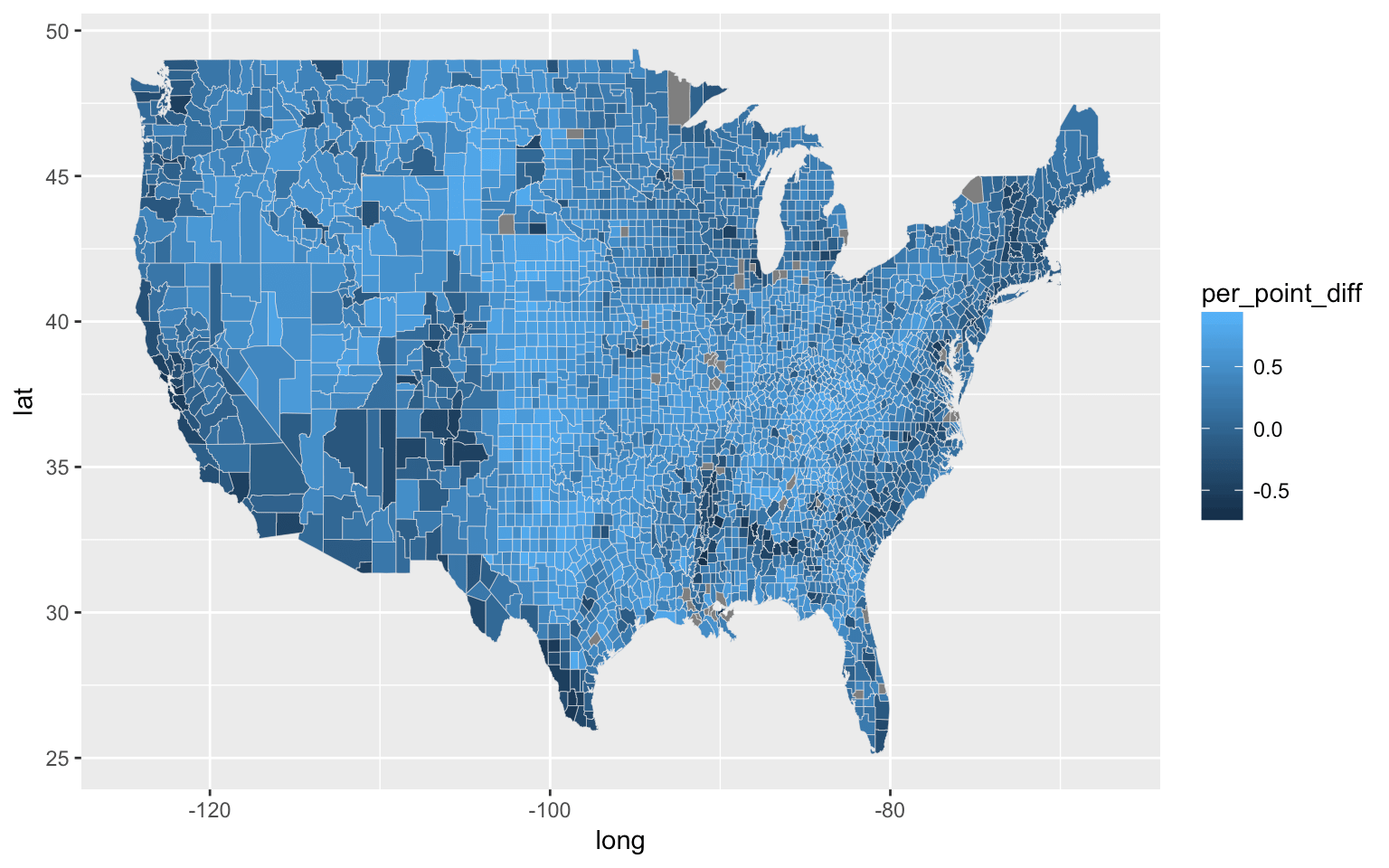Now, we fill the map with red and blue colors for the republican and democratic voting counties:

`cnty_plot <- cnty_plot + scale_fill_gradient2(midpoint = 0, low = "#2062A1", mid = "white", high = "red", na.value = "white", breaks = seq(from = 0, to = 1, length.out = 7), guide = FALSE)`

Generating this: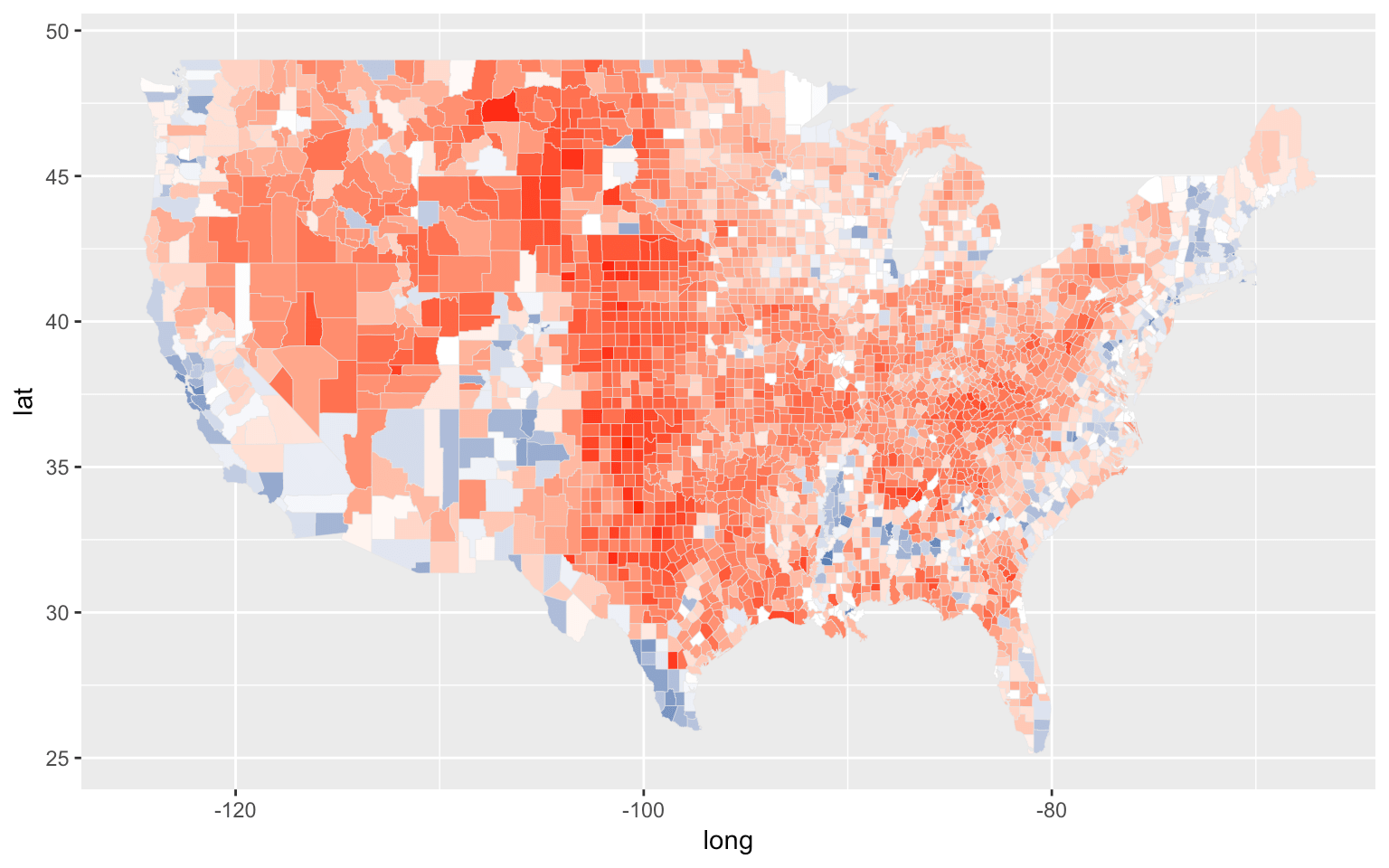Let’s remove all the distractions and add the store locations by zipcode:

`cnty_plot <- cnty_plot + theme_void() + geom_point(data = locationzips_df, aes(x = longitude, y = latitude), size = 0.3, alpha = 0.2, inherit.aes = FALSE)`

Giving us this: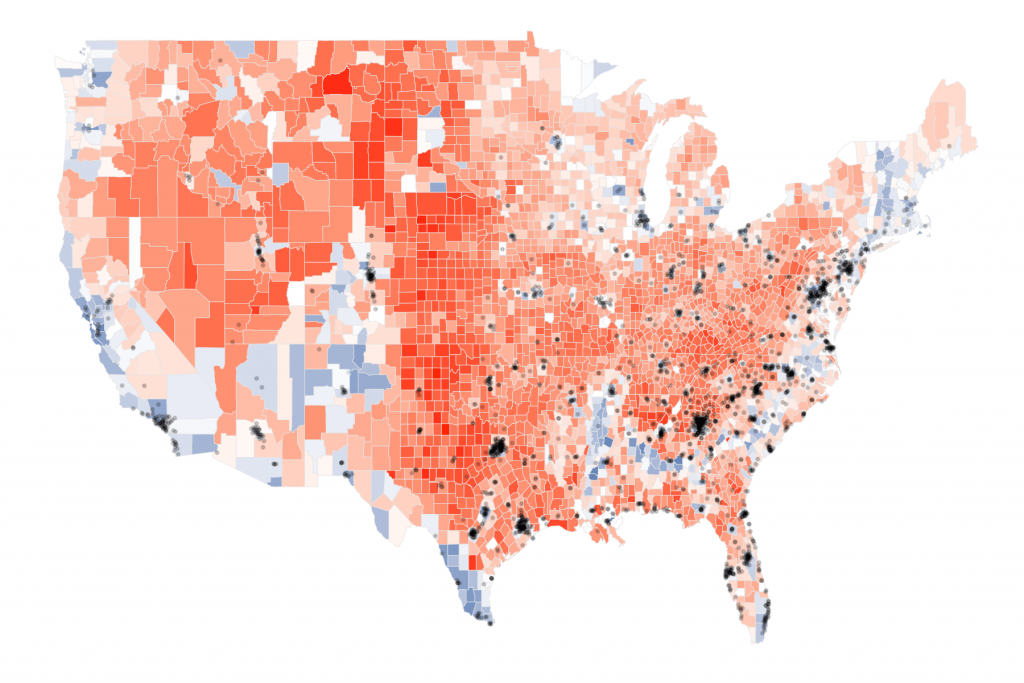Very different picture, wouldn’t you say? It actually looks like that the store locations are present in more democratic leaning counties or at least the counties that are equally divided between the republican and democratic votes.

Of course, it is possible that I messed something up. But, I can conclude two things based on this chart:

• Aggregation can mislead us to see the non-existent patterns
• A person’s political identification or views has nothing to do with the food he or she likes. And, the Chick-fil-A leaders know that.

What do you think?

# Complete Script

```library(rvest) library(stringr) library(dplyr) library(ggplot2) library(scales) library(ggmap) library(readr) library(tidyr) library(zipcode) library(maps) library(jsonlite) library(grid) library(gridExtra) data(zipcode) ## load zipcode data in your workspace   states <- read_html("https://www.Chick-fil-A.com/Locations/Browse")   locations <- states %>% html_nodes("article ul li a") %>% html_attr("href")   locations <- paste0("https://www.Chick-fil-A.com", locations) extract_location <- function(url){ read_html(url) %>% html_nodes(".location p") %>% html_text() %>% str_extract("\\d{5}(?=\n)") }   locationzips <- sapply(locations, extract_location, USE.NAMES = FALSE) locationzips_df <- data.frame(zips = unlist(locationzips), stringsAsFactors = FALSE)   #locationzips_df <- read_csv("https://www.dropbox.com/s/x8xwdx61go07e4e/chickfilalocationzips.csv?raw=1", col_types = "c")   locationzips_df\$zips <- clean.zipcodes(locationzips_df\$zips) locationzips_df <- merge(locationzips_df, select(zipcode, zip, latitude, longitude, state), by.x = 'zips', by.y = 'zip', all.x = TRUE)   est_cnt_by_state <- count(locationzips_df, state) head(rest_cnt_by_state)   region_opinion_favor <- fromJSON("http://ava.prri.org/ajx_map.regionsdata?category=lgbt_ssm&sc=2&year=2015&topic=lgbt")\$regions region_opinion_oppose <- fromJSON("http://ava.prri.org/ajx_map.regionsdata?category=lgbt_ssm&sc=3&year=2015&topic=lgbt")\$regions   region_opinion_favor\$opi <- 'favor' region_opinion_oppose\$opi <- 'oppose'   region_opinion <- bind_rows(region_opinion_favor, region_opinion_oppose) %>% filter(region != 'national') %>% mutate(region = recode(region, "1" = "Northeast", "2" = "Midwest", "3" = "South", "4" = "West")) %>% spread(key = opi, value = percent) %>% mutate(other = 100 - favor - oppose) %>% gather(key = opi, value = percent, -region, -sort) %>% select(-sort) %>% mutate(opi = factor(opi, levels = c('oppose', 'other', 'favor'), ordered = TRUE)) head(region_opinion)   regions_oppose_sorted <- arrange(filter(region_opinion, opi == 'oppose'), desc(percent))\$region region_opinion <- mutate(region_opinion, region = factor(region, levels = regions_oppose_sorted, ordered = TRUE))   opin_pie_charts <- ggplot(region_opinion, aes(x = 1, y = percent, fill = opi)) + geom_bar(width = 1,stat = "identity", size = 0.3, color = "white", alpha = 0.85, show.legend = FALSE) plot(opin_pie_charts) opin_pie_charts <- opin_pie_charts + facet_grid(region ~ ., switch = "y") + coord_polar("y") + theme_void() plot(opin_pie_charts) opin_pie_charts <- opin_pie_charts + scale_fill_manual(values = c("favor" = "black", "oppose" = "#908E89", "other" = "#F7F3E8")) plot(opin_pie_charts)   #add labels opin_pie_charts <- opin_pie_charts + geom_text(color = "white", size = rel(3.5), aes(x = 1, y = ifelse(opi == 'favor', 15, 85), label = ifelse(opi == 'other', NA, paste0(str_to_title(opi), "\n", percent(percent/100))))) plot(opin_pie_charts) opin_pie_charts <- opin_pie_charts + ggtitle(label = "Survey of views of gay\nmarriage, by region") + theme(plot.title = element_text(size = 10, face = "bold", hjust = 1)) plot(opin_pie_charts) opin_pie_charts <- opin_pie_charts + theme(strip.background = element_rect(fill = "#E6D9B7"), strip.text = element_text(face = "bold"), plot.background = element_rect(fill = "#F6F4E8", color = NA), panel.background = element_rect(fill = "#F6F4E8", color = NA)) plot(opin_pie_charts)   state_centers_abb <- data.frame(state = tolower(state.name), stateabr = state.abb, center_long = state.center\$x, center_lat = state.center\$y, region = state.region) head(state_centers_abb)   state_centers_abb <- mutate(state_centers_abb, region = recode(region, "North Central" = "Midwest"))   us_map <- map_data("state") us_map <- rename(us_map, state = region) us_map <- inner_join(us_map, state_centers_abb, by = c("state" = "state")) us_map <- us_map[order(us_map\$order), ]   base_plot <- ggplot(data = left_join(us_map, rest_cnt_by_state, by = c("stateabr" = "state")), aes(x = long, y = lat, group = group, order = order, fill = region)) + geom_polygon(color = "white") plot(base_plot)   base_plot <- base_plot + scale_fill_manual(values = c("West" = "#E6D9B7", "South" = "#F2DBD5", "Northeast" = "#D8E7D7", "Midwest" = "#D6DBE3"), guide = FALSE) plot(base_plot)   base_plot <- base_plot + theme(axis.title = element_blank(), axis.ticks = element_blank(), axis.line = element_blank(), axis.text = element_blank(), panel.grid = element_blank(), panel.border = element_blank(), plot.background = element_rect(fill = "#F6F4E8"), panel.background = element_rect(fill = "#F6F4E8")) plot(base_plot)   base_plot <- base_plot + geom_point(aes(size = n, x = center_long, y = center_lat), shape = 1, stroke = 0.5, color = "grey60", inherit.aes = FALSE) + scale_size_area(max_size = 18, breaks = c(0, 10, 100, 300), guide = FALSE) + scale_shape(solid = FALSE) plot(base_plot)   base_plot <- base_plot + annotation_custom(grob = circleGrob(r = unit(1.4, "lines"), gp = gpar(fill = "gray87", alpha = 0.5)), xmin = -79, xmax = -77, ymin = 48 , ymax = 49) + annotation_custom(grob = circleGrob(r = unit(0.7, "lines"), gp = gpar(fill = "gray85", alpha = 0.87)), xmin = -79, xmax = -77, ymin = 47.4, ymax = 48) + annotation_custom(grob = circleGrob(r = unit(0.3, "lines"), gp = gpar(fill = "gray80", alpha = 0.95)), xmin = -79, xmax = -77, ymin = 46.5, ymax = 48) plot(base_plot)   base_plot <- base_plot + ggtitle(label = "Number of Chick-fil-A resturants by state and region") + theme(plot.title = element_text(size = 10, face = "bold", hjust = 0.14, vjust = -5)) png("wsj-Chick-fil-A-data-visualization-r-plot.png", width = 800, height = 500, units = "px") grid.arrange(base_plot, opin_pie_charts, widths= c(4, 1), nrow = 1) dev.off()   cnty_results <- read_csv("https://raw.githubusercontent.com/tonmcg/County_Level_Election_Results_12-16/master/2016_US_County_Level_Presidential_Results.csv") cnty_results <- mutate(cnty_results, per_point_diff = per_gop - per_dem, county_name = tolower(str_replace(county_name, " County", "")), county_name = tolower(str_replace(county_name, " parish", "")))   county_map <- map_data("county") county_map <- rename(county_map, state = region, county = subregion) county_map\$stateabr <- state.abb[match(county_map\$state, tolower(state.name))] cnty_map_results <- left_join(county_map, cnty_results, by = c("stateabr" = "state_abbr", "county" = "county_name")) %>% arrange(order, group) cnty_plot <- ggplot(data = cnty_map_results, aes(x = long, y = lat, group = group, order = order)) + geom_polygon(aes(fill = per_point_diff), color = "gray90", size = 0.1) plot(cnty_plot)   cnty_plot <- cnty_plot + scale_fill_gradient2(midpoint = 0, low = "#2062A1", mid = "white", high = "red", na.value = "white", breaks = seq(from = 0, to = 1, length.out = 7), guide = FALSE) plot(cnty_plot)   cnty_plot <- cnty_plot + theme_void() + geom_point(data = locationzips_df, aes(x = longitude, y = latitude), size = 0.3, alpha = 0.2, inherit.aes = FALSE)```

A co-author of Data Science for Fundraising, an award winning keynote speaker, Ashutosh R. Nandeshwar is one of the few analytics professionals in the higher education industry who has developed analytical solutions for all stages of the student life cycle (from recruitment to giving). He enjoys speaking about the power of data, as well as ranting about data professionals who chase after “interesting” things. He earned his PhD/MS from West Virginia University and his BEng from Nagpur University, all in industrial engineering. Currently, he is leading the data science, reporting, and prospect development efforts at the University of Southern California.

•Andres says:

I have this error:

region_opinion_favor <- fromJSON("http://ava.publicreligion.org/ajx_map.regionsdata?category=lgbt_ssm&sc=2&year=2015&topic=lgbt&quot;)\$regions
Error in parse_con(txt, bigint_as_char) :
lexical error: invalid char in json text.
<!–[if IE 8
(right here) ——^

•Jas says:

Awesome work! However, I get an error when running:

region_opinion_favor <- fromJSON("http://ava.publicreligion.org/ajx_map.regionsdata?category=lgbt_ssm&sc=2&year=2015&topic=lgbt&quot;)\$regions

Error in parse_con(txt, bigint_as_char) :
lexical error: invalid char in json text.
<!–[if IE 8
(right here) ——^

Any tips?

•D says:

Hello! Thank you for sharing.

However, I am also having the same issue that others have commented on.

I am unable to run:

region_opinion_favor <- fromJSON("http://ava.publicreligion.org/ajx_map.regionsdata?category=lgbt_ssm&sc=2&year=2015&topic=lgbt&quot;)\$regions

or

region_opinion_oppose <- fromJSON("http://ava.publicreligion.org/ajx_map.regionsdata?category=lgbt_ssm&sc=3&year=2015&topic=lgbt&quot;)\$regions

I would really love to know how to resolve this.

•n.ashutosh says:

Thanks for letting me know about the error. It looks like they changed the data source. I’ve updated the post. Here it is:
region_opinion_favor <- fromJSON("http://ava.prri.org/ajx_map.regionsdata?category=lgbt_ssm&sc=2&year=2015&topic=lgbt&quot;)\$regions
region_opinion_oppose <- fromJSON("http://ava.prri.org/ajx_map.regionsdata?category=lgbt_ssm&sc=3&year=2015&topic=lgbt&quot;)\$regions